# 【信号处理】基于matlab实现男女声识别

+关注继续查看

## 2 部分代码

function SF = SpaceFrequency(image)%计算空间频率%=============================================%空间频率反映了一幅图像空间域的总体活跃度%=============================================% clear all% [filename,pathname]=uigetfile('*.*','图像');% A=imread([pathname,filename]);A=image;%s=size(size(A));%if s(2)==3%   A=rgb2gray(A);%endA=double(A);[M,N]=size(A);sum1=0;sum2=0;%计算行频率for i=1:M    for j=2:N        w=A(i,j)-A(i,j-1);        sum1=sum1+w^2;    endendRF=sqrt(sum1/(M*N));%计算列频率for j=1:N    for i=2:M        w=A(i,j)-A(i-1,j);        sum2=sum2+w^2;    endendCF=sqrt(sum2/(M*N));SF = sqrt(RF^2+CF^2);

## 3 仿真结果编辑编辑编辑编辑

## 4 参考文献

张仕玲, 孙旭. 基于Matlab的图像清晰度评价方法研究[J]. 通信技术, 2011, 44(12):3.

## 5 代码下载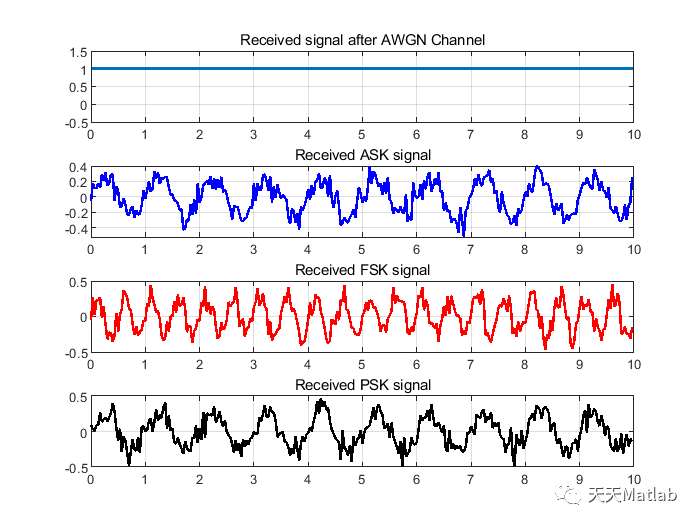0 0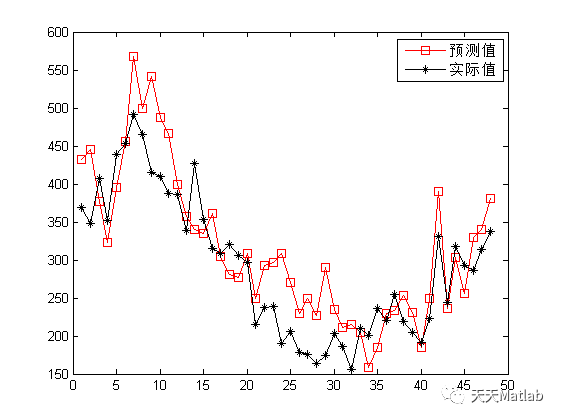【ELM回归预测】基于极限学习机实现气象影响因子光伏出力预测附matlab代码
【ELM回归预测】基于极限学习机实现气象影响因子光伏出力预测附matlab代码
0 0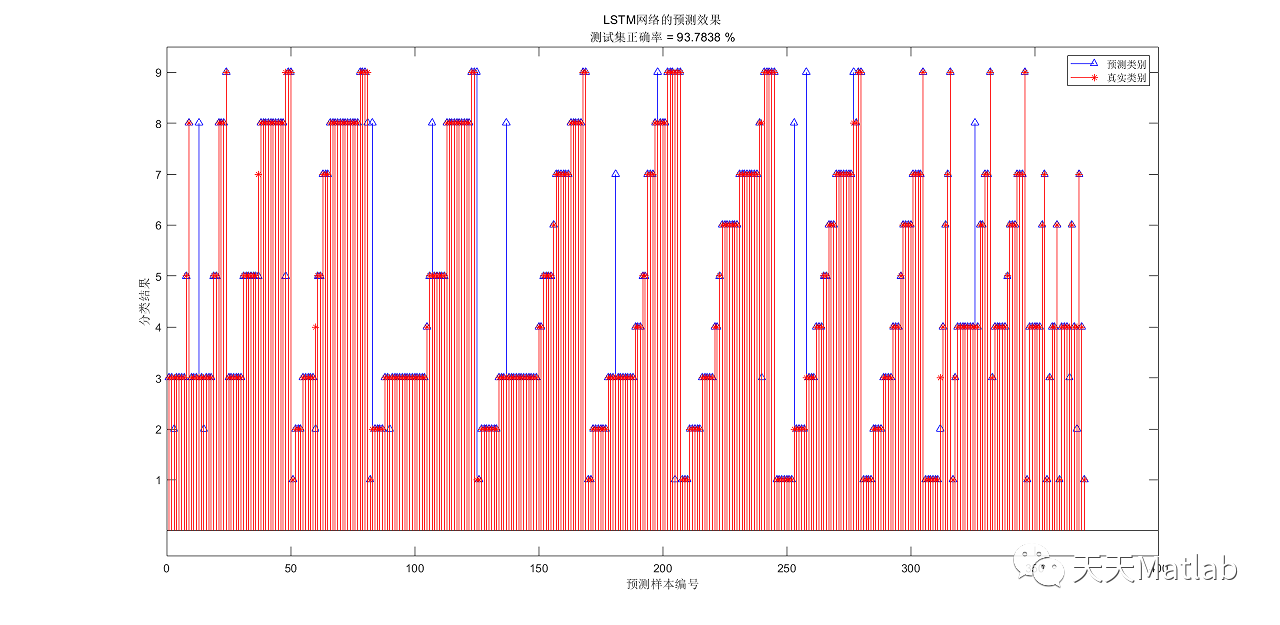0 0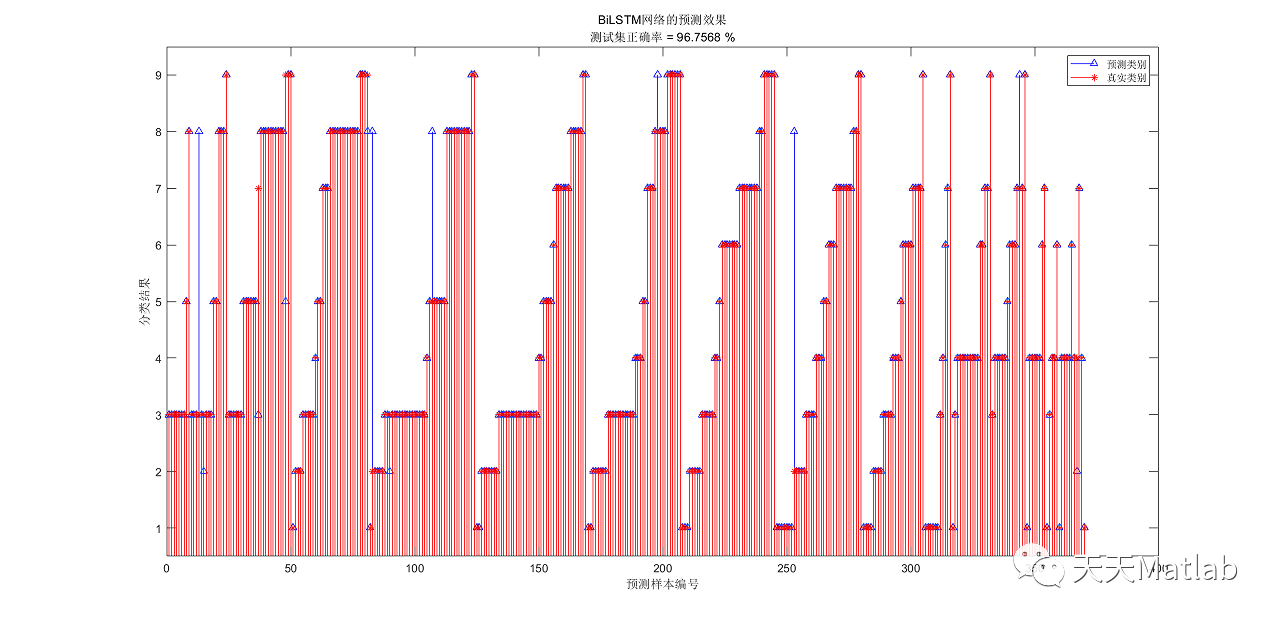0 0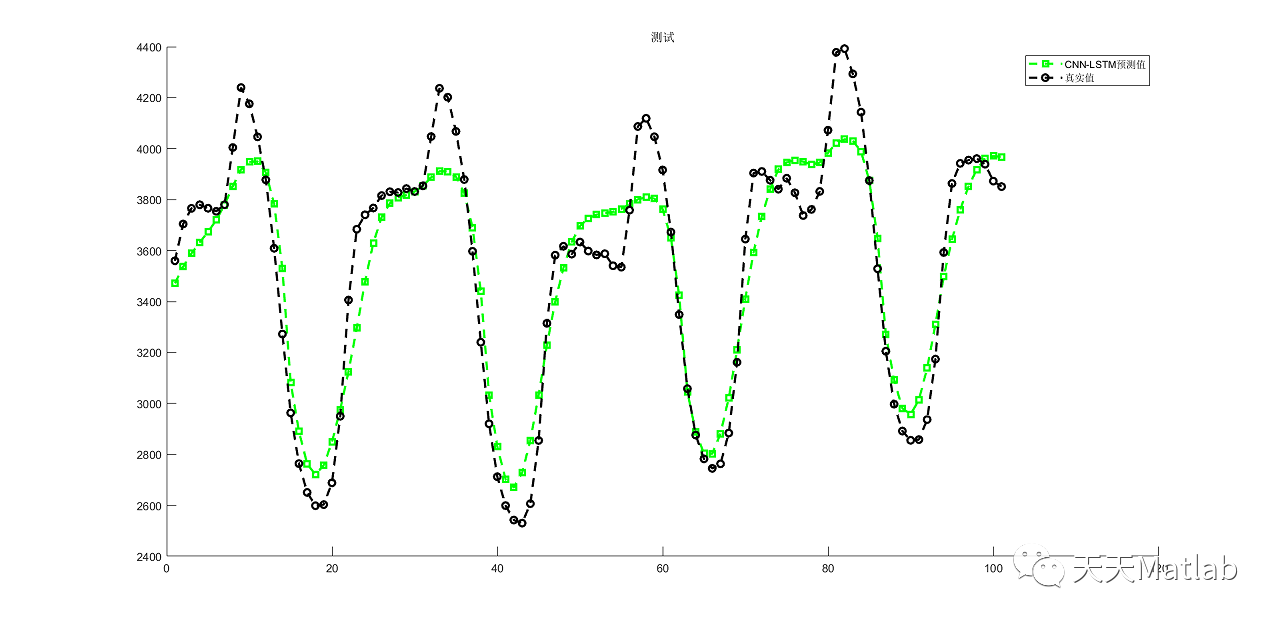【LSTM时序预测】基于卷积神经网络结合长短时记忆CNN-LSTM实现时序数据预测附matlab代码
【LSTM时序预测】基于卷积神经网络结合长短时记忆CNN-LSTM实现时序数据预测附matlab代码
0 0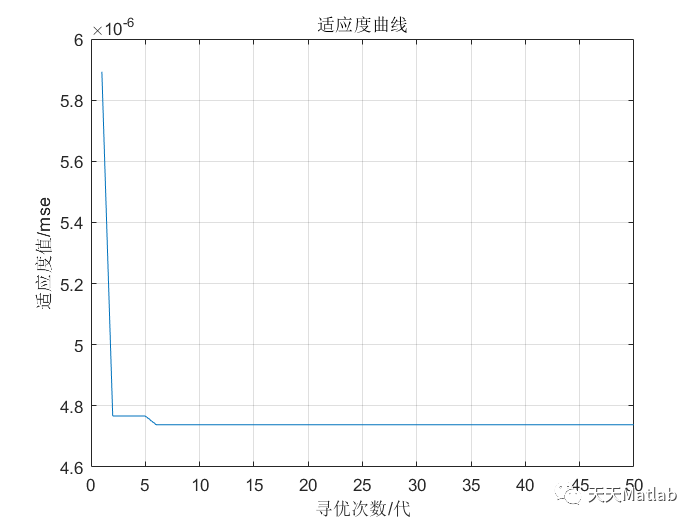【Kelm回归预测】基于粒子群算法优化核极限学习机实现数据回归预测附matlab代码
【Kelm回归预测】基于粒子群算法优化核极限学习机实现数据回归预测附matlab代码
0 0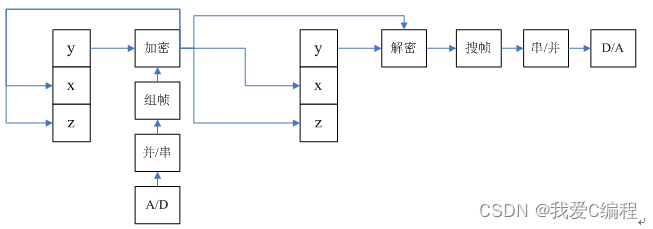m基于Lorenz混沌自同步的混沌数字保密通信系统的FPGA实现,verilog编程实现+MATLAB混沌验证程序
m基于Lorenz混沌自同步的混沌数字保密通信系统的FPGA实现,verilog编程实现+MATLAB混沌验证程序
0 0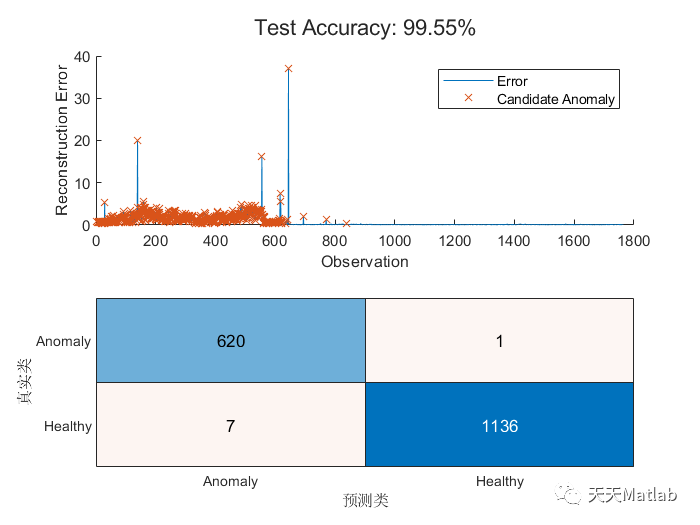【信号检测】基于LSTM实现工业机器信号数据异常检测附matlab代码
【信号检测】基于LSTM实现工业机器信号数据异常检测附matlab代码
0 0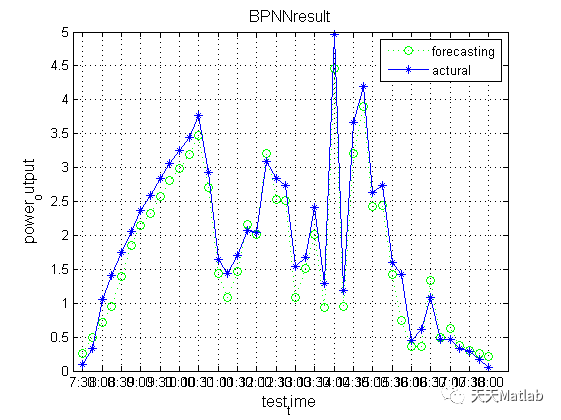【BP回归预测】基于遗传算法优化BP神经网络GA-BP实现光伏出力预测附matlab代码
【BP回归预测】基于遗传算法优化BP神经网络GA-BP实现光伏出力预测附matlab代码
0 0
Matlab科研工作室